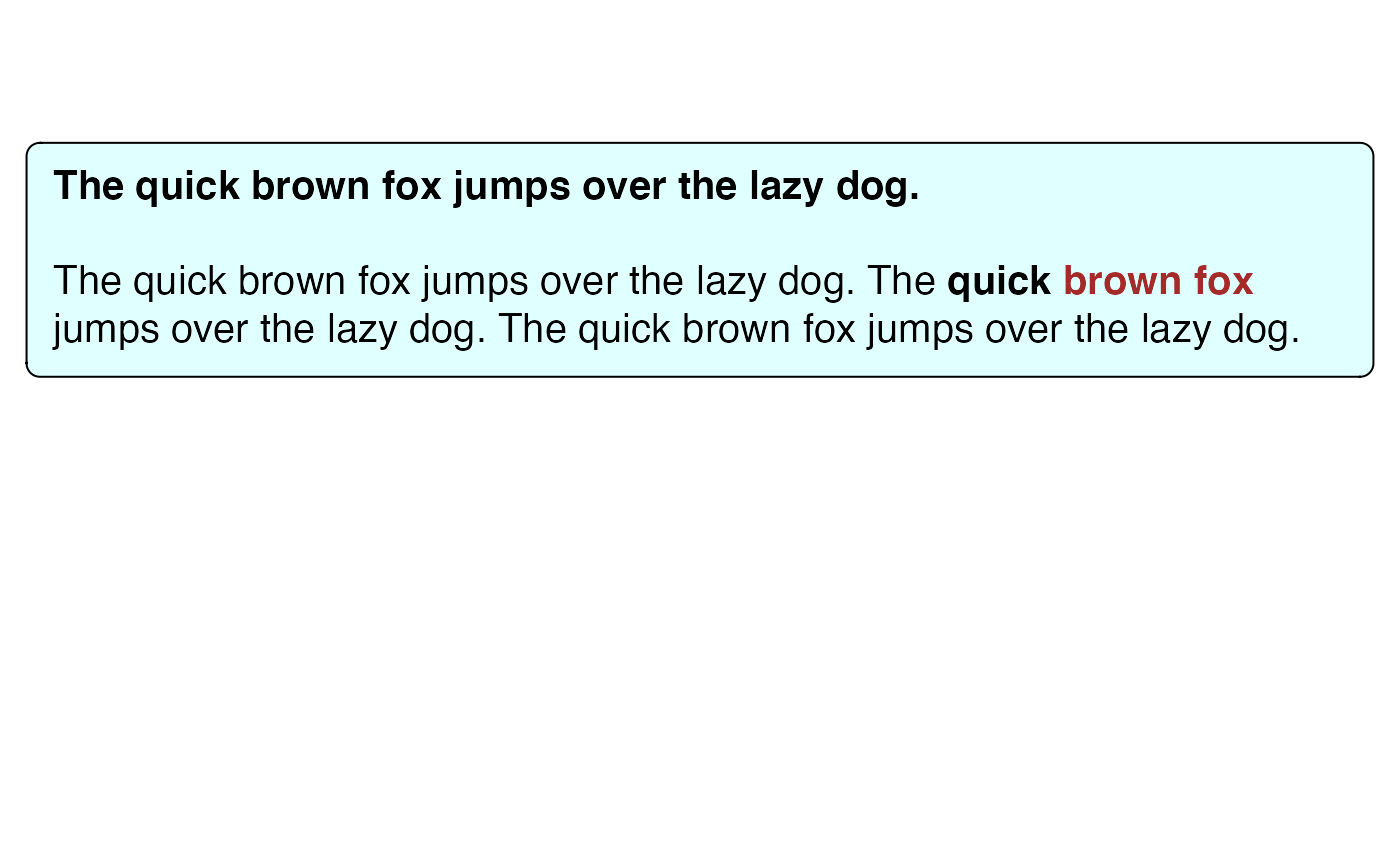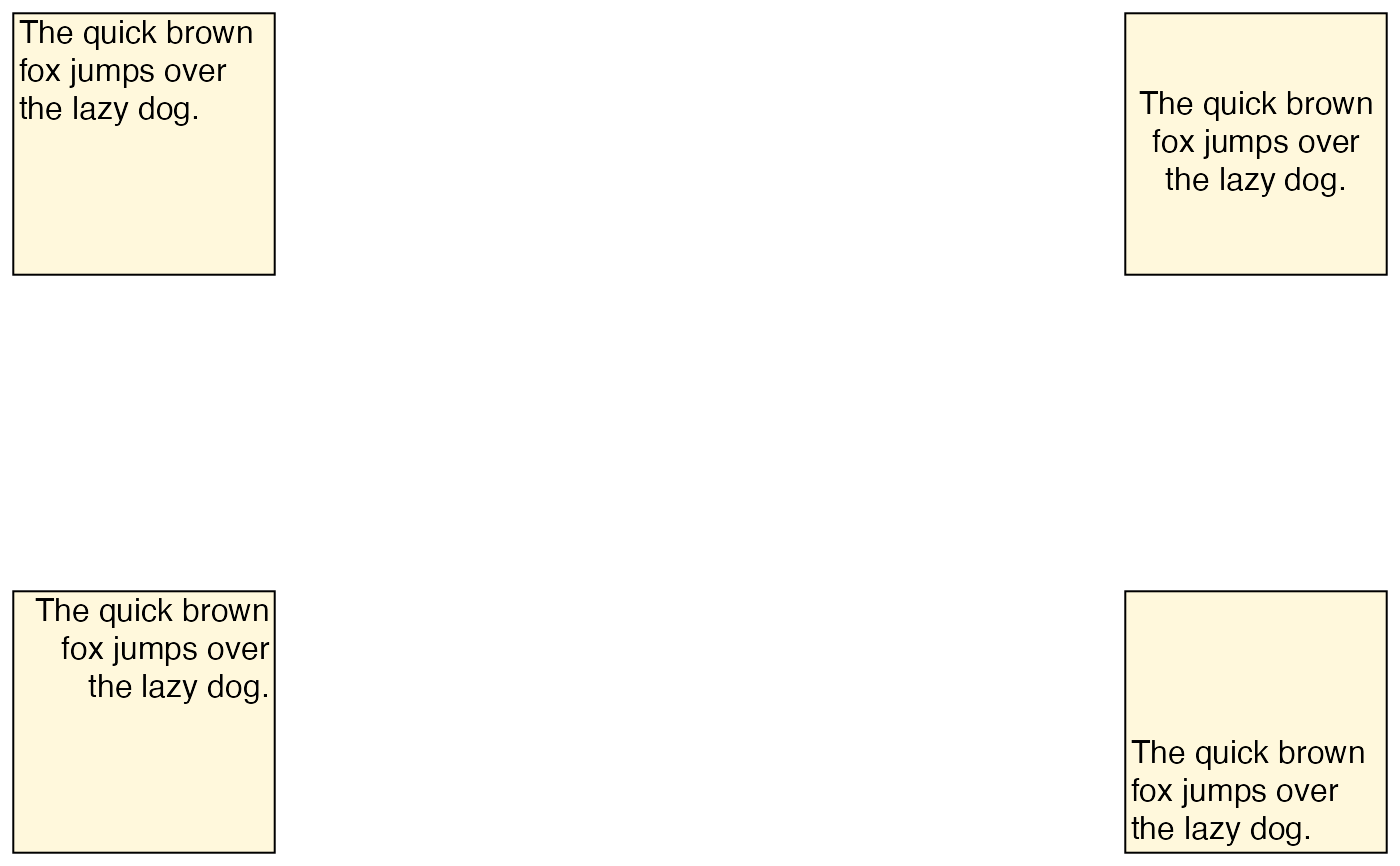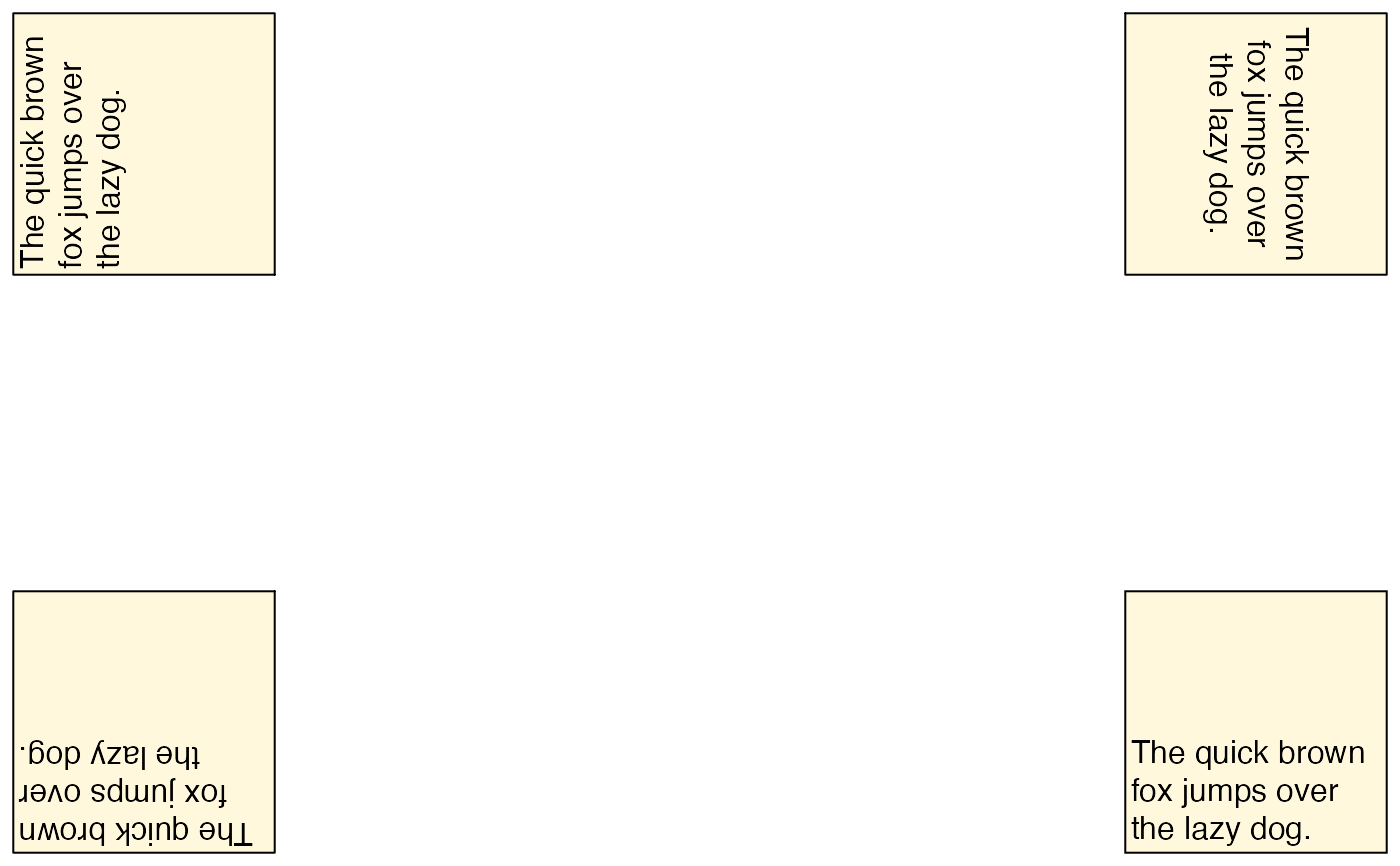The function textbox_grob() is intended to render multi-line text labels that require automatic word wrapping. It is similar to richtext_grob(), but there are a few important differences. First, while richtext_grob() is vectorized, textbox_grob() is not. It can draw only a single text box at a time. Second, textbox_grob() doesn't support rendering the text box at arbitrary angles. Only four different orientations are supported, corresponding to a rotation by 0, 90, 180, and 270 degrees.

textbox_grob(
text,
x = NULL,
y = NULL,
width = unit(1, "npc"),
height = NULL,
minwidth = NULL,
maxwidth = NULL,
minheight = NULL,
maxheight = NULL,
hjust = 0.5,
vjust = 0.5,
halign = 0,
valign = 1,
default.units = "npc",
margin = unit(c(0, 0, 0, 0), "pt"),
padding = unit(c(0, 0, 0, 0), "pt"),
r = unit(0, "pt"),
orientation = c("upright", "left-rotated", "right-rotated", "inverted"),
name = NULL,
gp = gpar(),
box_gp = gpar(col = NA),
vp = NULL,
use_markdown = TRUE
)

## Arguments

text Character vector containing Markdown/HTML string to draw. Unit objects specifying the location of the reference point. If set to NULL (the default), these values are chosen based on the values of hjust and vjust such that the box is appropriately justified in the enclosing viewport. Unit objects specifying width and height of the grob. A value of NULL means take up exactly the space necessary to render all content. Use a value of unit(1, "npc") to have the box take up all available space. Min and max values for width and height. Set to NULL to impose neither a minimum nor a maximum. Note: minheight and maxheight do not work if width = NULL. Numerical values specifying the justification of the text box relative to the reference point defined by x and y. These justification parameters are specified in the internal reference frame of the text box, so that, for example, hjust adjusts the vertical justification when the text box is left- or right-rotated. Numerical values specifying the justification of the text inside the text box. Units of x, y, width, height, minwidth, minheight, maxwidth, maxheight if these are provided only as numerical values. Unit vectors of four elements each indicating the margin and padding around each text label in the order top, right, bottom, left. Margins are drawn outside the enclosing box (if any), and padding is drawn inside. To avoid rendering artifacts, it is best to specify these values in absolute units (such as points, mm, or inch) rather than in relative units (such as npc). The radius of the rounded corners. To avoid rendering artifacts, it is best to specify this in absolute units (such as points, mm, or inch) rather than in relative units (such as npc). Orientation of the box. Allowed values are "upright", "left-rotated", "right-rotated", and "inverted", corresponding to a rotation by 0, 90, 270, and 180 degrees counter-clockwise, respectively. Name of the grob. Other graphical parameters for drawing. Graphical parameters for the enclosing box around each text label. Viewport. Should the text input be treated as markdown?

## Value

A grid grob that represents the formatted text.

richtext_grob()

## Examples

library(grid)
g <- textbox_grob(
"**The quick brown fox jumps over the lazy dog.**<br><br>
The quick brown fox jumps over the lazy dog.
The **quick <span style='color:brown;'>brown fox</span>** jumps over the lazy dog.
The quick brown fox jumps over the lazy dog.",
x = unit(0.5, "npc"), y = unit(0.7, "npc"), halign = 0, valign = 1,
gp = gpar(fontsize = 15),
box_gp = gpar(col = "black", fill = "lightcyan1"),
r = unit(5, "pt"),
padding = unit(c(10, 10, 10, 10), "pt"),
margin = unit(c(0, 10, 0, 10), "pt")
)
grid.newpage()
grid.draw(g)# internal vs. external alignment
g1 <- textbox_grob(
"The quick brown fox jumps over the lazy dog.",
hjust = 0, vjust = 1, halign = 0, valign = 1,
width = unit(1.5, "inch"), height = unit(1.5, "inch"),
box_gp = gpar(col = "black", fill = "cornsilk"),
padding = unit(c(2, 2, 2, 2), "pt"),
margin = unit(c(5, 5, 5, 5), "pt")
)
g2 <- textbox_grob(
"The quick brown fox jumps over the lazy dog.",
hjust = 1, vjust = 1, halign = 0.5, valign = 0.5,
width = unit(1.5, "inch"), height = unit(1.5, "inch"),
box_gp = gpar(col = "black", fill = "cornsilk"),
padding = unit(c(2, 2, 2, 2), "pt"),
margin = unit(c(5, 5, 5, 5), "pt")
)
g3 <- textbox_grob(
"The quick brown fox jumps over the lazy dog.",
hjust = 0, vjust = 0, halign = 1, valign = 1,
width = unit(1.5, "inch"), height = unit(1.5, "inch"),
box_gp = gpar(col = "black", fill = "cornsilk"),
padding = unit(c(2, 2, 2, 2), "pt"),
margin = unit(c(5, 5, 5, 5), "pt")
)
g4 <- textbox_grob(
"The quick brown fox jumps over the lazy dog.",
hjust = 1, vjust = 0, halign = 0, valign = 0,
width = unit(1.5, "inch"), height = unit(1.5, "inch"),
box_gp = gpar(col = "black", fill = "cornsilk"),
padding = unit(c(2, 2, 2, 2), "pt"),
margin = unit(c(5, 5, 5, 5), "pt")
)
grid.newpage()
grid.draw(g1)
grid.draw(g2)
grid.draw(g3)
grid.draw(g4)# internal vs. external alignment, with rotated boxes
g1 <- textbox_grob(
"The quick brown fox jumps over the lazy dog.",
hjust = 1, vjust = 1, halign = 0, valign = 1,
width = unit(1.5, "inch"), height = unit(1.5, "inch"),
orientation = "left-rotated",
box_gp = gpar(col = "black", fill = "cornsilk"),
padding = unit(c(2, 2, 2, 2), "pt"),
margin = unit(c(5, 5, 5, 5), "pt")
)
g2 <- textbox_grob(
"The quick brown fox jumps over the lazy dog.",
hjust = 0, vjust = 1, halign = 0.5, valign = 0.5,
width = unit(1.5, "inch"), height = unit(1.5, "inch"),
orientation = "right-rotated",
box_gp = gpar(col = "black", fill = "cornsilk"),
padding = unit(c(2, 2, 2, 2), "pt"),
margin = unit(c(5, 5, 5, 5), "pt")
)
g3 <- textbox_grob(
"The quick brown fox jumps over the lazy dog.",
hjust = 1, vjust = 1, halign = 1, valign = 1,
width = unit(1.5, "inch"), height = unit(1.5, "inch"),
orientation = "inverted",
box_gp = gpar(col = "black", fill = "cornsilk"),
padding = unit(c(2, 2, 2, 2), "pt"),
margin = unit(c(5, 5, 5, 5), "pt")
)
g4 <- textbox_grob(
"The quick brown fox jumps over the lazy dog.",
hjust = 1, vjust = 0, halign = 0, valign = 0,
width = unit(1.5, "inch"), height = unit(1.5, "inch"),
orientation = "upright",
box_gp = gpar(col = "black", fill = "cornsilk"),
padding = unit(c(2, 2, 2, 2), "pt"),
margin = unit(c(5, 5, 5, 5), "pt")
)
grid.newpage()
grid.draw(g1)
grid.draw(g2)
grid.draw(g3)
grid.draw(g4)